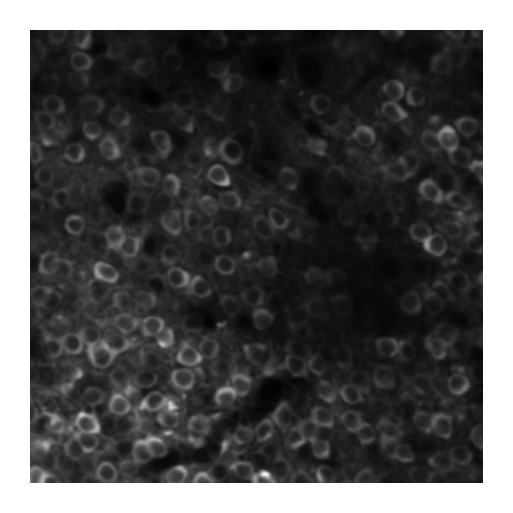In :
import numpy as np
import time, os, sys
from urllib.parse import urlparse
import matplotlib.pyplot as plt
import matplotlib as mpl
%matplotlib inline
mpl.rcParams['figure.dpi'] = 300
from cellpose import utils, io

urls = ['http://www.cellpose.org/static/images/img02.png',
'http://www.cellpose.org/static/images/img03.png',
'http://www.cellpose.org/static/images/img05.png']
files = []
for url in urls:
parts = urlparse(url)
filename = os.path.basename(parts.path)
if not os.path.exists(filename):
files.append(filename)

# REPLACE FILES WITH YOUR IMAGE PATHS
# files = ['img0.tif', 'img1.tif']

# view 1 image
plt.figure(figsize=(2,2))
plt.imshow(img)
plt.axis('off')
plt.show()In :
# RUN CELLPOSE

from cellpose import models, io

# DEFINE CELLPOSE MODEL
# model_type='cyto' or model_type='nuclei'
model = models.Cellpose(gpu=False, model_type='cyto')

# define CHANNELS to run segementation on
# grayscale=0, R=1, G=2, B=3
# channels = [cytoplasm, nucleus]
# if NUCLEUS channel does not exist, set the second channel to 0
# channels = [0,0]
# IF ALL YOUR IMAGES ARE THE SAME TYPE, you can give a list with 2 elements
# channels = [0,0] # IF YOU HAVE GRAYSCALE
# channels = [2,3] # IF YOU HAVE G=cytoplasm and B=nucleus
# channels = [2,1] # IF YOU HAVE G=cytoplasm and R=nucleus

# or if you have different types of channels in each image
channels = [[2,3], [0,0], [0,0]]

# if diameter is set to None, the size of the cells is estimated on a per image basis
# you can set the average cell diameter in pixels yourself (recommended)
# diameter can be a list or a single number for all images

# you can run all in a list e.g.
# >>> imgs = [io.imread(filename) in for filename in files]
# >>> masks, flows, styles, diams = model.eval(imgs, diameter=None, channels=channels)
# >>> io.save_to_png(imgs, masks, flows, files)

# or in a loop
for chan, filename in zip(channels, files):
masks, flows, styles, diams = model.eval(img, diameter=None, channels=chan)

# save results so you can load in gui

# save results as png

>>>> using CPU
Running test snippet to check if MKL-DNN working
see https://pytorch.org/docs/stable/backends.html?highlight=mkl
** MKL version working - CPU version is sped up. **
processing 1 image(s)
computing styles from images
time spent: running network 2.57s; flow+mask computation 0.75
estimated cell diameters for 1 image(s) in 5.28 sec
>>> diameter(s) =  [29.38115358]
time spent: running network 10.96s; flow+mask computation 1.21
estimated masks for 1 image(s) in 12.18 sec
>>>> TOTAL TIME 17.46 sec
processing 1 image(s)
computing styles from images
time spent: running network 2.25s; flow+mask computation 0.62
estimated cell diameters for 1 image(s) in 5.07 sec
>>> diameter(s) =  [33.17679957]
time spent: running network 8.61s; flow+mask computation 0.72
estimated masks for 1 image(s) in 9.34 sec
>>>> TOTAL TIME 14.41 sec
processing 1 image(s)
computing styles from images
time spent: running network 8.84s; flow+mask computation 1.99
estimated cell diameters for 1 image(s) in 16.19 sec
>>> diameter(s) =  [30.58094729]
time spent: running network 22.57s; flow+mask computation 2.26
estimated masks for 1 image(s) in 24.84 sec
>>>> TOTAL TIME 41.04 sec

In :
# DISPLAY RESULTS
from cellpose import plot

fig = plt.figure(figsize=(12,5))